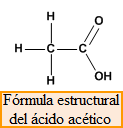The empirical formula indicates the atoms that participate in the compound as well as their proportion. For example, glucose has the empirical formula CH 2 O, which indicates the presence of carbon, oxygen and hydrogen in its structure in a 1:2:1 ratio. However, the actual formula of the glucose molecule is C 6 H 12 O 6.

Molecular formula , it is the real formula of the molecule, it indicates the types of atoms and the number of each type that participate in the formation of the molecule. For example, the molecular formula for glucose, C 6 H 12 O 6 , tells us that each molecule is made up of 6 C atoms, 12 hydrogen atoms, and 6 oxygen atoms.Structural formula , shows the way in which the different atoms are joined to give rise to the molecule. For example, acetic acid has the molecular formula, C 2 H 4 O 2 , which does not indicate how the 8 atoms that make up the molecule are joined. The structural formula shows us that one of the carbons is attached by single bonds to three hydrogens and the second carbon. For its part, the second carbon forms a double bond with the first oxygen and a single bond with the second, which in turn joins a hydrogen.Finally, we must consider that molecules have a spatial arrangement, they are three-dimensional structures, and we must use molecular models to represent them satisfactorily. A widely used model is the ball-and-bar model shown below.